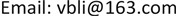1. 引言

2. 图的半监督学习理论

w i j = { exp ( − ‖ x i − x j ‖ 2 σ ) ,   if     x i ≠ x j 0                   otherwise (1)

L * ( f ) = min { ∑ i = 1 l L ( f ( x i ) , y i ) } (3)

s ( f ) = f ′ L f = ∑ i , j = 1 n w i j ( f ( x i ) d i − f ( x j ) d j ) 2 (4)

f * = arg min f ( x i ) { s + γ L * } = arg min f ( x i ) { f ′ L f + γ ∑ i = 1 l L ( f ( x i ) , y i ) } (5)

3. 基于优化的半监督学习的行人检测算法3.1. 特征提取和行人候选区域选取

(6)

3.2. 优化图的半监督学习模型的构建3.2.1. 构建优化图的半监督行人检测模型

min ∑ i = 1 l + ∑ j = 1 l + w i , j (7)

max ∑ i = 1 l + ∑ j = l + l − w i , j (8)

W = arg min w i , j ∑ i = 1 l ∑ j = 1 l h i , j w i , j (9)

h i j = { 1 ,     if   1 ≤ i , j ≤ l + − 1 ,     if   1 ≤ i ≤ l + ;   l + < j ≤ l − 0 ,   otherwise (10)

3.2.2. 优化图的半监督行人检测模型的分析

min ω , b ∑ i = 1 l ∑ j = 1 l ( w ′ i , j − 〈 ω ⋅ x i , j 〉 − b ) 2 (12)

min w , b       1 2 ‖ ω ‖ 2 s .t .         ( ( ω ⋅ x i , j ) + b ) − w ′ i , j ≤ ε , i , j = 1 , ⋯ , l               w ′ i , j − ( ( ω ⋅ x i , j ) + b ) ≤ ε , i , j = 1 , ⋯ , l (13)

L ( ω , b , α ( * ) ) = 1 2 ‖ ω ‖ 2 − ∑ i = 1 l ∑ j = 1 l α i , j ( ε + w ′ i , j − ( ω ⋅ x i , j ) − b )                 − ∑ i = 1 l ∑ j = 1 l α i , j * ( ε − w ′ i , j + ( ω ⋅ x i , j ) + b ) (14)

min a , a ( * )     1 2 ∑ i = 1 l ∑ j = 1 l ∑ m = 1 l ∑ n = 1 l ( a i , j * − a i , j ) ( a m , n * − a m , n ) ( x i , j ⋅ x m , n ) − ε ∑ i , j = 1 l ( a i , j * + a i , j ) s .t .   ∑ i = 1 , j l ( a i , j − a i , j * ) = 0 ,     a i , j ( * ) ≥ 0 , i , j = 1 , ⋯ , l (16)

w ″ ( x i , j ) = ∑ i = 1 l ∑ j = 1 l ( α i , j * − α i , j ) ( x ⋅ x i , j ) + b (17)

(19)

4. 实验结果及分析

(20)

precision = N c N c + N f (21)

Experiment Comparison with SC + GLS
RecallPrecision
Our method0.7620.613
SC+GLSS0.7190.497

Experiment Comparison with HOG + SV
RecallPrecision
Our method0.7640.613
HOG+SVM0.7340.560

5. 结束语